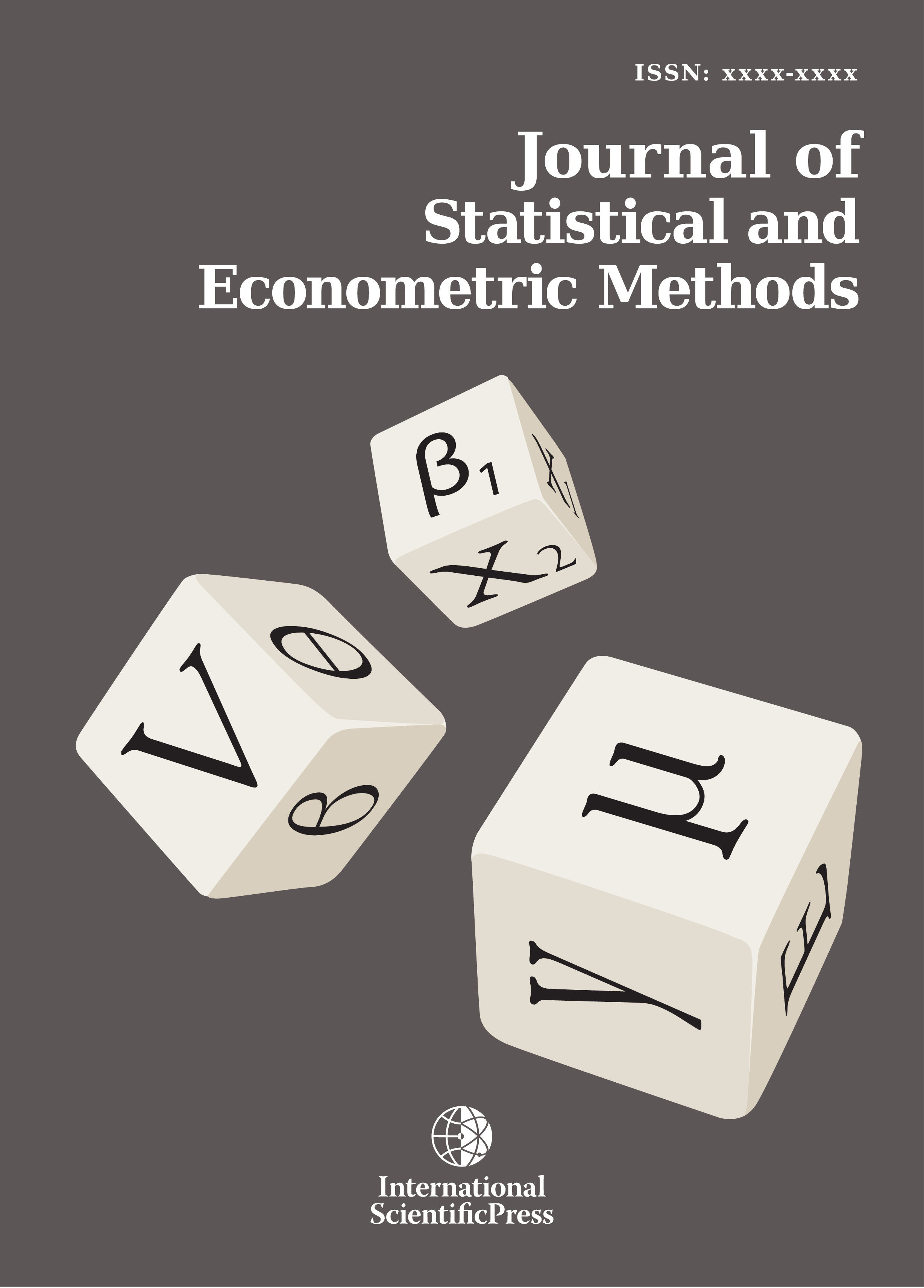# Journal of Statistical and Econometric Methods

#### Estimation of Nested Error Non-parametric Unit Level Model

•[ Download ]
• Times downloaded: 201
• Abstract

In this paper, we proposed a nested error nonparametric unit level model, when the linearity assumptions have been violated. The model formulation and parameter estimation of mean function were examined and proposed two theorems for asymptotic properties of mean function of proposed model were done. The simulation study was performed and it has shown that the mean square errors (MSE) and bias of our estimated were close to zero.

Keywords: Schwarzs inequality; Triangle inequalityISSN: 2241-0376 (Online)
2241-0384 (Print)